# Plotting integers on a number line Online Quiz

#### Complete Python Prime Pack

9 Courses     2 eBooks

#### Artificial Intelligence & Machine Learning Prime Pack

6 Courses     1 eBooks

#### Java Prime Pack

9 Courses     2 eBooks

Following quiz provides Multiple Choice Questions (MCQs) related to Plotting integers on a number line. You will have to read all the given answers and click over the correct answer. If you are not sure about the answer then you can check the answer using Show Answer button. You can use Next Quiz button to check new set of questions in the quiz.Q 1 - Which letter best represents −22?### Explanation

Step 1:

−22 lies to the right of −52 on the given number line.

Step 2:

From the image of given number line, B best represents −22 as −55 < −22.

Q 2 - Which letter best represents −19?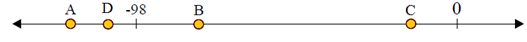### Explanation

Step 1:

−19 lies to the right of −98 on the given number

Step 2:

From the image of given number line, C best represents −19 as −98 < −19.

Q 3 - Which letter best represents −91?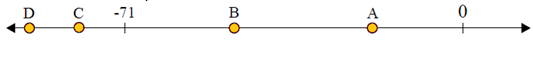### Explanation

Step 1:

−91 lies to the left of −71 on the given number line.

Step 2:

From the image of given number line, D best represents −91 as −91 < −71.

Q 4 - Which letter best represents 27?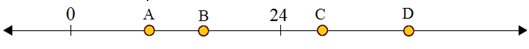### Explanation

Step 1:

27 lies to the right of 24 on the given number line.

Step 2:

From the image of given number line, C best represents 27 as 24 < 27.

Q 5 - Which letter best represents 32?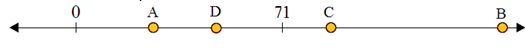### Explanation

Step 1:

32 lies to the left of 71 on the given number line.

Step 2:

From the image of given number line, A best represents −32 as 32 < 71.

Q 6 - Which letter best represents 114?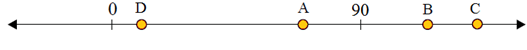### Explanation

Step 1:

114 lies to the right of 90 on the given number line.

Step 2:

From the image of given number line, B best represents 114 as 90 < 114.

Q 7 - Which letter best represents 29?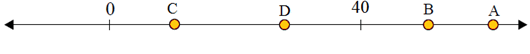### Explanation

Step 1:

29 lies to the left of 40 on the given number line.

Step 2:

From the image of given number line, D best represents 29 as 29 < 40.

Q 8 - Which letter best represents −16?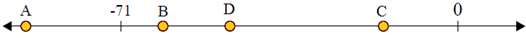### Explanation

Step 1:

−16 lies to the right of −71 on the given number line.

Step 2:

From the image of given number line, C best represents −16 as −71 < −16.

Q 9 - Which letter best represents −58?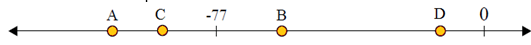### Explanation

Step 1:

−58 lies to the right of −77 on the given number line.

Step 2:

From the image of given number line, B best represents −58 as −77 < −58.

Q 10 - Which letter best represents −32?### Explanation

Step 1:

−32 lies to the right of −78 on the given number line.

Step 2:

From the image of given number line, D best represents −32 as −78 < −32.

plotting_integers_on_number_line.htm